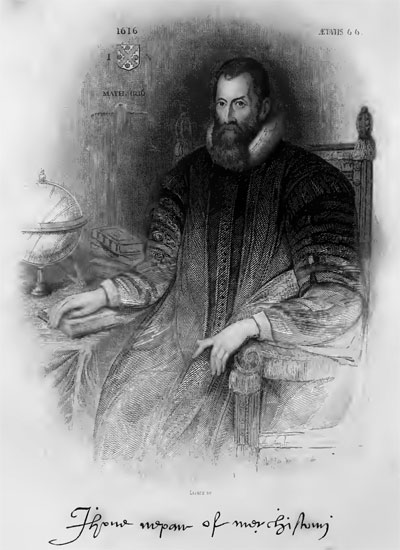# Napier's Bones

Seeing there is nothing that is so troublesome to mathematical practice… than the multiplications, divisions, square and cubical extractions of great numbers, which besides the tedious expense of time are... subject to many slippery errors, I began therefore to consider [how] I might remove those hindrances.
Excerpt from A Description of the Wonderful Canon of Logarithms

***

In 1617 in Edinburgh after the death of John Napier (see biography of John Napier) was published (in Latin language, which was a common practice then) his small book —Rabdologiae seu Numerationis per Virgulas libri duo (see the photo below). Looking to ease his own difficulties in calculating logarithmic tables, and impatient with the tedious and error-prone process of working with large numbers, Napier invented several mechanical methods of simplifying and speeding up multiplication, the most famous being special rods, later known as Napier's bones. Besides the virgulas or rods, John Napier described multiplicationes promptuario (promptuary of multiplication or lightning calculator), and the scacchiae abaco or chessboard abacus.

Napier published a description of his inventions in Rabdologiae, the title of which he derived from the Greek ραβδoς (rod) and λόγος (word) (incidentally, this section of Napier's work also contains the first printed reference to the decimal point). The reason for publishing the work is given by Napier in the dedication, where he says that so many of his friends, to whom he had shown the numbering rods, were so pleased with them that they were already becoming widely used, even beginning to be used in foreign countries (one of these friends was Alexander Seton, the Earl of Dumfermline and High Chancellor of Scotland).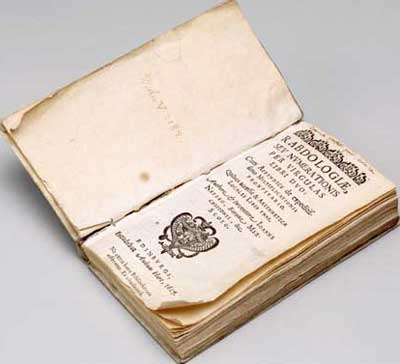As opposed to the title ...libri duo (two books), actually the Rabdologiae consists of four parts (books), two basic, and two appendixes. Book I is a description of the calculating tool, Book II offers forty-seven pages of tables, examples, and general problems demonstrating the utility of the rods in solving questions of geometry and mechanics. Book III is an appendix on Napier's promptuary, a more elaborate calculating device consisting of engraved rods and strips; and Book IV is an appendix of forty-one pages, devoted to so called arithmeticæ localis (location arithmetic).

Napier apparently based his invention on a popular in this time method for multiplication, described in several books—e.g. in the book of the famous Italian mathematician Luca Paccioli Summa de Arithmetica, Geometrica, Proportioni et Proportionalita (Everything about Arithmetic, Geometry, and Proportion), printed in Venice in 1494. This method was called gelosia (jealousy) in Italian. Most probably this ancient methods for multiplication was invented by the Indian mathematicians, then was transferred to China and via Arabian Halifat—to Europe. The gelosia method is as follows:

A grid of squares, divided into parts by a diagonal, must be cross-ruled, as the number of squares depends on the number of digits in factors, e.g. if we want to multiply 3-digital to 3-digital factor, then the grid must be 3x3 squares. To the upper side and right side of the grid must be written the 2 factors, and intermediate products are written in the squares in such manner, that the diagonal divides the units from the tens. The units of the partial product (the digit from the right by the digit from the upper) are written on one side and the tens on the other, so that when a multiple consists of two figures they are separated by the diagonal. To get the final product, the numbers along the diagonals are added and the result is written to the left of grid (senior digital positions) and below the grid (junior digital positions).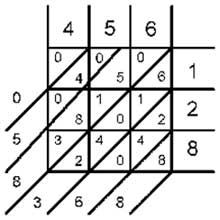Let's see an example, to multiply 456x128 (see the nearby figure).
As the two numbers are 3-positional, we have to draw 3x3 grid, to the upper side we have to write 456 (first factor) and to the right side 128 (second factor). In the squares, divided by a diagonal, we have to write the products of a digit placed to the upper side of a particular column to the digit, placed to the right side, as in upper left part of the square we have to write tens (if any), while in the lower right part we have to write units. Then we have to prolong the diagonals and to add digits in every diagonal, starting with the units and if it necessary, we make a carry to the next diagonal. In such manner we get the result—456x128=058368. The multiplication was done by means of addition.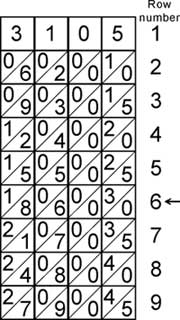What Napier did (how often simple things are of genius!?), is that he made slips (columns) with all possible 9 columns of squares of the gelosia grid, and thus he can put aside manual drawing of a grid and writing in squares. These slips are written on the surface of ten rods, later on called Napier's rods (the best sets of Napier's numbering rods were made of ivory, so that they looked like bones, which explains why they are now known as Napier's bones).

Let's make a multiplication by means of Napier's rods, e.g. 3105 x 6 (see nearby figure). We arrange side by side four rods (for 3, 1, 0 and 5).
First we have to take the row for the factor 6 (marked with arrow). We start from the right, taking initially zero from the lower right part of the first cell. It will be the first digit of the result. Then we have to add the three digits from the left part of the first cell with 0 from the right part of the second cell. Continuing to add the digits along the diagonals and we will get the proper result 18630.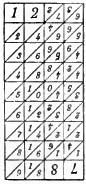During the multiplication of a number, which has identical digits, we have to use identical rods. That's why Napier suggested rods to take the form of a parallelepiped, on the four surfaces of which to be inscribed four digital columns of rods in such manner, that the four faces of each rod to contain multiples of one of the nine digits, and is similar to one of the slips just described, the first rod containing the multiples of 0, 1, 9, 8, the second of 0, 2. 9, 7, the third of 0, 3, 9, 6, the fourth of 0, 4, 9, 5, the fifth of 1, 2, 8 , 7 (see the nearby figure), the sixth of 1, 3, 8, 6, the seventh of 1, 4, 8, 5, the eighth of 2, 3, 7, 6, the ninth of 2, 4, 7, 5, and the tenth of 3, 4, 6, 5. Each rod, therefore, contains on two of its faces factors of digits which are complementary to those on the other two faces, and the factors of a digit and its complement are reversed in position.

When the second factor is multidigital, then intermediate products must be written manually, shifting one position leftwards, then intermediate products must be added (see the drawing below). After arranging rods for the multiplicand side by side, we have to multiply the multiplicand (46785399) by the units of the multiplier (96431). This the result is 46785399x1=46785399. Then we have to multiply the multiplicand by the tens the multiplier 46785399x3=140356197, shifting the result one position left and to continue, while all digits of the multiplier will be used. Then we have to add manually the partial factors. It was a matter of time, someone to think about, that if we have an adding machine, the multiplication can be done without any thinking, and this happened only some years later—Wilhelm Schickard, who used Napier's Rods in his Rechenuhr (Calculating Clock).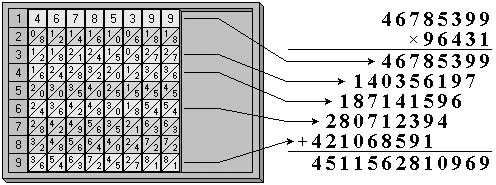Multiplication of multidigital numbers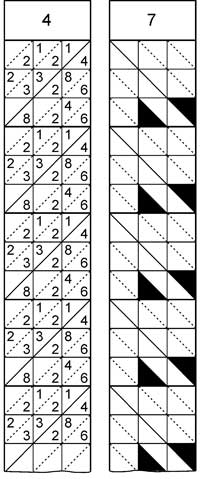Obviously, the use of Napier's rods is easy, but tedious when one wants to multiply two numbers each having two or more digits. That's why Napier went further, and in the book III of his Rabdologiae he described a more elaborate calculating device, consisting of engraved rods and strips (so called promptuary). Promptuary comes from the Latin promptuarium, "a place where things are stored ready for use".
The device consists of 200 (100 thick and 100 thin) rods, placed in a special box—promptuary (one set consists of 10 rods for every digit). The tick rod is 5 mm tick, while the thin is 2,5 mm. Side surface of each rod is divided into ten squares and two rectangles (in the upper and lower part of the surface), in which are inscribed digits from 0 to 9. The squares are divided into triangles: these of thick rods—with diagonals from the upper right corner to the left lower, while the squares of the thin rods—from the left upper to the right lower (on the nearby figure you can see part from two rods, to the left is placed the thick rod for the digit 4, to the right is placed thin rod for the 7, cut off (missing) triangles are filled with black color).
Tick rods are filled with digits (or empty for zero), while on the surface of thin rods are cut off triangle openings (without openings for zero). Squares of the rods, which are inscribes with digits from 1-9 (not 0), are divided into 9 smaller squares, and every of these squares is divided in half into 3 triangles by means of a diagonal, parallel to the diagonal in the bigger square. In the triangles are inscribed digits according to the letters a, b, c, d, e, f, g, i, j, as shown in the lower figure, following the rule:

On the surface of the thick rods are inscribed multiple numbers of digits, marked on the rod, as the digit of the rod is inscribed instead of letter a, doubled digit is inscribed instead of letter b and so on. If the multiple number has two digits, then tens are inscribed instead of the upper letters, while the units are inscribed instead of appropriate lower letter (see the lower figure).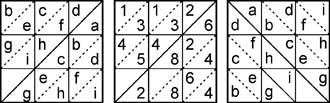Left square is inscribed with letters for the thick rod. The middle square is inscribed with letters for the rod for 6. Right square is for letters of the thin rod.

On the surface of thin rod with header 1, the openings are cut off at the place of triangles, marked with the letter a, on the surface of thin rod with header 2, the openings are cut off at the place of triangles, marked with the letter b and so on (as it is shown in the right square of the upper figure).

During the multiplication the thick rod is leaned against the thin rod in such manner, that the diagonals of the two big squares to coincide (thin rods are rotated to 90 degrees). Then in the openings of the thin rod can be seen the digits from the product of the numbers, which are inscribed on these rods. In the next figure you can see the multiplication 7213x524. First must be arranged properly thick rods, according to the multiplicand 7213. Then the thin rods, according to the multiplier 524, are placed over and rotated to the 90 degrees to the thick, as the diagonals of squares on the thick and thin rods to be concurrent. The result can be shown by adding of visible digits (in the figure the digits are shown in the thicken triangles).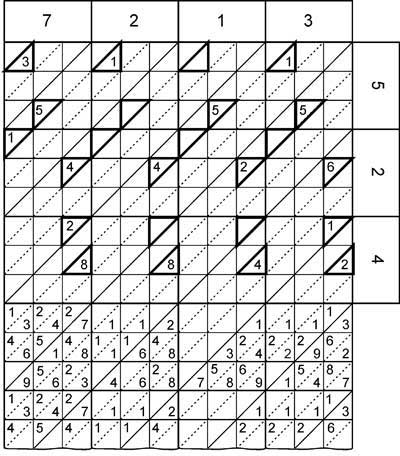Multiplication 7213x524 with promptuary rods

In the description of the promptuary Napier specified, that it can be used for division also. For this action he suggest to be found first the reciprocal of one of the multipliers, then to be done a multiplication with the promptuary.

Napier's rods rapidly became popular in England. According to one Seth Partridge, a London-based surveyor and mathematical practitioner, these reckoning rods were easy to make in any material whatsoever; they could either be manufactured by oneself or bought in the London instrument shops:
These speaking-Rods may be made either of Silver, Brasse, Ivorie, or Wood, as the maker and user of them best pleaseth, but they are most ordinarily made of good sollid Box, and being thereof made, they are as usefull as those made of any other substance whatsoever, Nay, I hold them more light and nimble then those made of Mettall; …Every practitioner may make them himselfe by cutting the faces of every one of the printed papers of the Rods, and so placed on a square piece of wood as before; or else they are ready made in Wood, by Master John Thompson…

In the next centuries a lot of inventors tried to improve and facilitate the work with Napier's rods, starting with the above mentioned Wilhelm Schickard in early 1620s.

In early 1650s, an attempt to make a tool with Napier's rods made the French physicist, cartographer, and engineer Pierre Petit (1594-1677), a King Counsellor and Intendant des Fortifications. Petit placed paper strips with Napier's rods and made a mechanism, the so called Arithmetical Cylinder or Tambour de Petit (Cylinder of Petit) (see the figure below), allowing the paper strips to be moved along the axes. The device he described in his book Dissertations academiques sur la nature du froid et du chaud. Avec un Discourssur la construction & l'usage d'un Cylindre Arithmetique, inventé par le mesme Autheur (Paris, 1653).The Arithmetical Cylinder of Pierre Petit

According to Petit, people ceased using Napier's "beautiful invention" because "the multitude and embarrassment of those sticks, filled with numbers on all sides, proved prolonged and tedious." Since Petit found this method of calculating still useful, and because it was "easier to improve on inventions than to become an inventor", he designed long bands or ribbons of paper each containing all the multiples of Napier's rabdology. Those long bands were then attached end to end and mounted on a wooden cylinder the size of a child's drum or a hat, and of a length which depended on the quantity of bands one wished to have in order to make calculations with large numbers.

The reckoning principles were identical to Napier's bones. Pierre Petit deemed these common enough by then that he wrote only a brief summary of how to proceed toward making a multiplication and a division.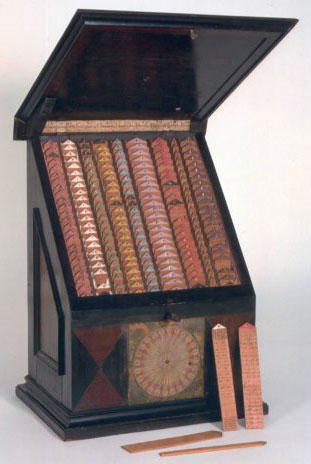Several years after Petit, in late 1650s, a device with Napier's rodes developed the famous German scientist Athanasius Kircher (1602-1680), who is mentioned in other articles of this site—for Kircher's automata and for Kircher's version of the Llullistic method.

In late 1650s as a prominent mathematician Kircher was asked to prepare a set of mathematical tools for teaching the young Austrian crown prince (Archduke) Karl Joseph Erzherzog von Österreich (1649-1664). The order was fulfilled and a set of ten different tools of bone-like tablets for performing a variety of different tasks was manufactured (see the nearby image of the tool, placed now in the Istituto e museo di storia della scienza, Florence, Italy) and sent to Archduke in 1661, who was very pleased. The toolset (called Organum Mathematicum, Mathematische Orgel or cista) was placed in a veneered wooden chest with a hinged lid (taking up the space of a large desk) and was described later in a book of his pupil and friend Gaspar Schott (1608-1666)—Organum Mathematicum libris IX explicatum, published in 1668 in Würzburg, Germany.

Schott not only described Kircher's Organum Mathematicum, but added his own improvement to Napier's reckoning rods (he most probably didn't know for the Rechenuhr of Schickard, created in early 1620s, which also used rods, places on a cylinder's surface). Instead of having to deal with a number of individual little rods each time one desired to perform multiplication, Schott designed a box (cistula) in which Napier's rods were converted into cylinders, each one of them incorporating the complete set of multiples from one to nine previously found on several separate rods. To operate the machine, one only had to turn the cylinders' handles to the proper figure needed to be multiplied, and it then only became a matter of following Napier's well-defined rules of rabdology. Moreover, to ensure the machine would be utilized by the greatest possible number of people, a table of addition and subtraction was provided on the inside cover of the box.

Later on the multiplication tool from organum mathematicum (with improvement of Schott) was described in Theatrum arithmetico-geometricum of Leupold. The arithmetic tool consists of ten cylinders, on which surface are placed strips with inscribed Napier's rods. The cylinders (see the lower figure) are mounted in a box, which is closed to the upper side from a lined sheet of pasteboard with narrow vertical slits. From the front side of the box are placed handles, attached to the cylinders, which can be used for rotating of the cylinders, thus can be set needed multiplicand on the rods. On the inner side of the hinged lid of the box is inscribed an addition table to aid the operator.The arithmetic tool of Organum Mathematicum in Theatrum arithmetico-geometricum of Leupold

In 1667 Sir Charles Cotterell (1615–1701), an English courtier and translator, devised a calculating instrument with Napier's rods (see arithmetical compendium of Cotterell), which included a wire-and-bead abacus for adding the partial products. The device was made around 1670 by the instrument maker Robert Jole in London.

In 1673 cylindre artihmetique (Napier's bones engraved on a cylinder) were used in the simple adding instrument nouvelle machine d'arithmétique of René Grillet de Roven.

In 1673 was published the book The Description and Use of Two Arithmetick Instruments of Samuel Morland. In this book are described two calculating devices, one of them, so called multiplying machine was based on Napier's rods. All calculating devices of Morland are described in the article for Morland in this site.

In the Theatrum arithmetico-geometricum of Leupold, already mentioned in this article, is described also a calculating tool, so called calculating drum, based on Napier's rods (see the lower figure). Lets examine its construction.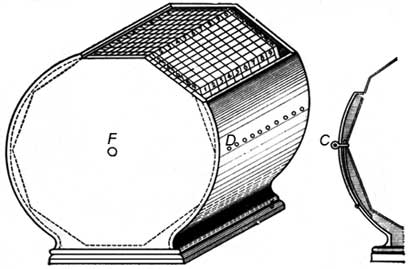Leupold's Napier's rods device (calculating drum)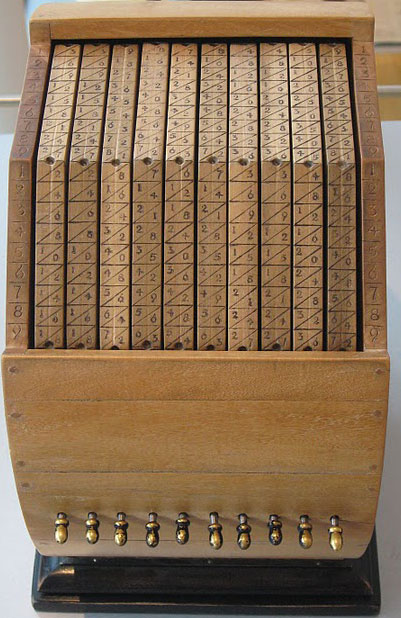The calculating drum of Leupold (in the nearby image you can a modern replica, from the exposition of Arithmeum Museum, Bonn) consists of 11 ten-sided disks, mounted on a common axes (marked with F in the drawing). The right side disk is fixed, while the remaining ten disks can be rotated with a hand. On the surface of the device are placed 10 round openings (marked with D), which are used for fixing the angular position of the disks, by means of pins (C), pushed in the appropriate opening, as it is shown in the right side of the figure. On the surface of each of the 10 sides of the rotating disk are inscribed the digits of the same Napier's rod, while on the side of the fixed disk, with face to the operator, is inscribed a column of digits from 1 to 9. The multiplicand is entered by rotating of the proper disks and fixing them by means of the pins against the fixed column of digits (multiplicands) of the fixed disk.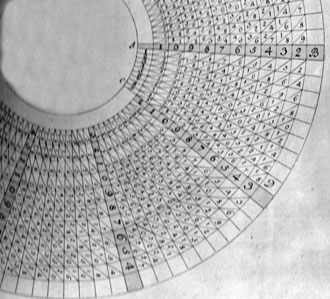In 1728 the German scientist Johann Michael Poetius described in his book "Anleitung zu[r] arithmetischen Wissenschaft, vermittelst einer parallelen Algebra" (Instructions for arithmetic means of science, a parallel algebra) an instrument, composed of concentric moving circles (so called Mensula Pythagorica), which seems to be a variation of the Napier's bones and can not render more services than the multiplication table (on the nearby image is shown a sector of Mensula Pythagorica).

In 1789, the German mathematician M. Prahl devised an instrument, which he called Arithmetica Portatilis, and which was the same as the Mensula Pythagorica of Poetius, except only that the movable circles were much larger and carried the numerals 1 to 100, so that with the aid of that instrument numbers could be added and subtracted up to 100.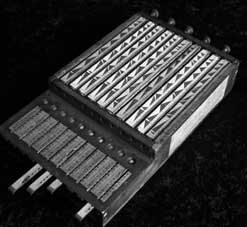One of the recent chapters in the development of Napier's bones as a calculating instrument took place in 1885, when the French mathematician—François Édouard Anatole Lucas (1842–1891) presented to the Académie Française a problem on arithmetic that caught the attention of Henri Genaille, a French civil engineer, employed by the railway system. Genaille, who was already quite well known for his invention of several different arithmetic aids, solved Lucas's problem and, in the process, devised a different form of Napier's rods. This instrument eliminated the need to carry digits from one column to the next when reading off partial products. Genaille demonstrated his instrument (so called Réglettes multiplicatrices or in English Genaille-Lucas rulers) in 1891. Lucas gave these rulers enough publicity that they became quite popular for a number of years and several instruments, based on the rulers have been manufactured (see the nearby photo).

The set of Genaille-Lucas rulers contains 11 strips. The physical size of the set is: the black cardboard container is 12 x 19 x 1 cm, the 11 rods each measure 1 x 1 x 17 cm. First strip (marked with Index in the lower figure) has only one useful side, which corresponds to the multiplier. It has nine rectangles (for the digits from 1 to 9), as the height of each rectangle is proportional to the digit in it. The remaining ten strips have four useful sides, as the each side of a particular strip is for different digit of the multiplicand. In the upper part of the strip is inscribed the digit of the multiplicand, and the lower part of the strip is divided into two vertical columns.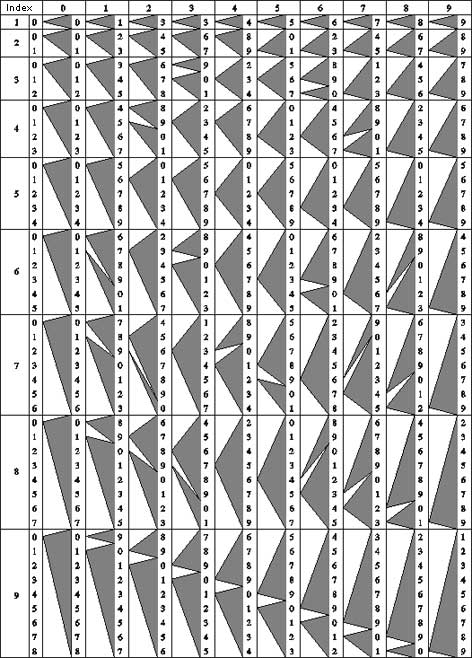Genaille-Lucas rulersThe multiplication can be done, as the strips for all digits of the multiplicand are arranged side by side, then the result can be read from right to the left (black triangles in the left column of the strip for each digit of the multiplicand), as first can be read units, then—tens and so on.
Let's make a simple multiplication with the rulers (3271x4) (see the nearby figure). First we have to arrange side by side the proper rulers for the multiplicand and index ruler, placing also to the left the ruler for 0. Then, starting with the fourth rectangle (this for the digit four) of the rightmost (index) ruler (the multiplier is 4) leftwards, we have to select the digit at the top of the rectangle (4 in this case) and then simply to follow the black arrows leftwards, reading off the digits as we come to them—4, 8, 0, 3, 1, and thus we have the product 13084. In contrast to Napier's rods, the result was obtained without making any arithmetical operations.
Henri Genaille created also a special set of rulers for division, which are similar to the multiplication rulers, except that the large arrows are replaced by a multitude of smaller ones.

Let's say some words about Book IV of Rabdologiae, which is an appendix of forty-one pages, devoted to so called arithmeticæ localis (location arithmetic). This appendix contains one of the first explorations of binary arithmetic as a computation aid. Location arithmetic is a technique to do binary arithmetic using a chessboard-like grid. Using simple moves of counters on the board, Napier showed ways to multiply, divide and even find the square roots of binary numbers. He was so pleased by his discovery that he said in the preface:
...it might be well described as more of a lark than a labor, for it carries out addition, subtraction, multiplication, division and the extraction of square roots purely by moving counters from place to place.

Location arithmetic uses a square grid where each square on the grid represents a value. Two sides of the grid are marked with increasing powers of two. Any inner square can be identified by two numbers on these two sides, one being vertically below the inner square and the other to its far right. The value of the square is the product of these two numbers. A very good description of location arithmetic can be found in the site of Mr. Stephan Weiss, www.mechrech.info.